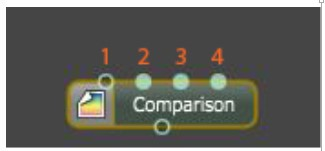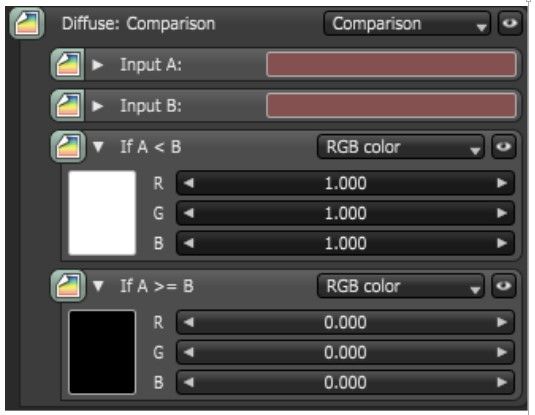# Comparison

The Comparison node offers the ability to use a logical comparison operator as a way to combine textures. The node takes four inputs: the first two inputs are the textures for comparison. The next two inputs are the result of the comparison.

In the following illustrations, the NodeGraph Editor window is used to clearly illustrate the association of the various texture nodes.Figure 1: The various input pins for the Comparison node: (1) Input 1, (2) Input 2, (3) If A<B, (4) If A>=B

This is a conditional Texture node for altering a given texture (input A) based on another texture (input B). In its simplest form, the Comparison node chooses between two alternative textures at render time. In the example below (figure 2), the result will be black since inputs A and B are the same, and it evaluates to true for the second condition.Figure 2: The same color for inputs A and B result in a black color